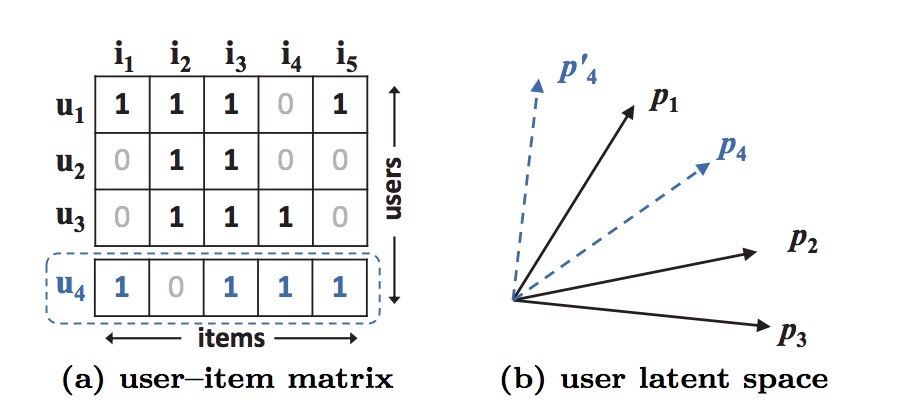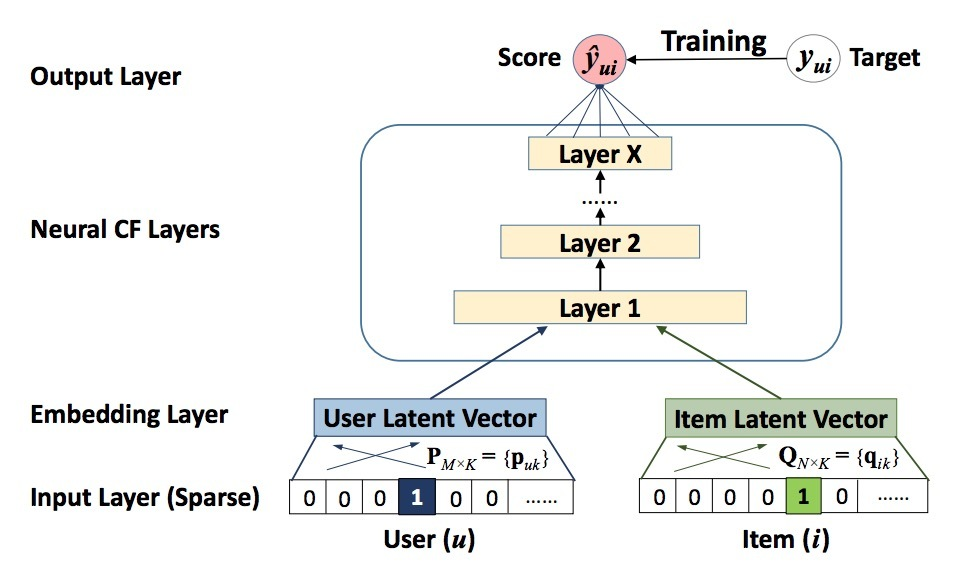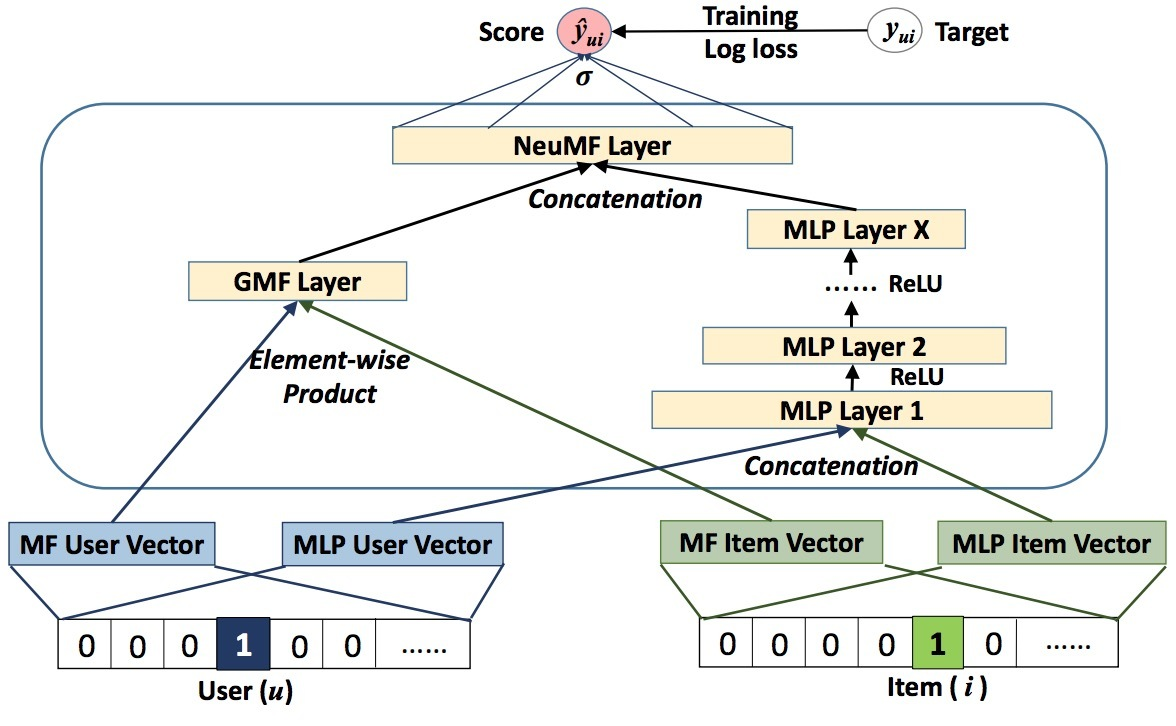2.前置

2.1 使用隐式数据进行学习

$y_{ui} = \begin{cases} 1, \\ 0 \end{cases}$

…(1)

$y_{ui}$为1表示：user u和item i间有交互；然而，它并不意味着u实际会喜欢i。相似的，该值为0也并不表示用户u不喜欢item i。对隐式数据的学习存在着许多挑战，因为它存在许多关于用户偏好的噪声数据。而已观察条目至少反映了用户在这些items上的兴趣，未观察条目可能只是缺失数据（missing data），它天然缺少负反馈

• 作为在显式反馈上的一个天然扩展，pointwise learning方法通常会使用一个回归框架，对$\hat{y}_{ui}$与它的目标值$y_{ui}$间的平方loss（squared loss）进行最小化。为了处理缺失的negative数据，会将所有未观察条目当成是负反馈，或者从未观察条目中进行抽样。
• pairwise learning的思想是：已观察条目应比未观察条目排序更靠前。pairwise learning会最大化已观察条目$\hat{y}_{ui}$与未观察条目$\hat{y}_{uj}$间的间隔。

2.2 矩阵分解（MF）

MF会分别将每个user和item使用一个关于隐特征的real-valued vector进行关联。假设：

• $p_u$和$q_i$表示user u和item i的隐向量

MF会将对$y_{ui}$的估计表示成$p_u$和$q_i$的内积：

$\hat{y}_{ui}=f(u,i|p_u,q_i) = p_u^T q_i = \sum\limits_{k=1}^{K} p_{uk} q_{ik}$

…(2)

MF会建模关于user和item隐因子的two-way interaction，假设隐空间的每个维度相互独立，并使用相同的权重进行线性组合。这样，MF可以被看成是一个关于隐因子的线性模型。

• 首先，由于MF会将users和items映射到相同的隐空间上，两个用户间的相似度可以通过一个内积、或者隐向量间夹角的cosine来进行度量。
• 第二，不失一般性，我们可以使用jaccard系数作为MF所需要去恢复的两个用户间的ground truth相似度。3. neural CF

3.1 通用框架$\hat{y}_{ui} = f(P^T v_u^U, Q^T v_i^I | P, Q, \theta_f)$

…(3)

$f(P^T v_u^U, Q^T v_i^I) = \phi_{out} (\phi_X (...\phi_2(\phi_1(P^T v_u^U, Q^T v_i^I))...))$

…(4)

3.1.1 NCF学习

$L_{sqr} = \sum_{(u,i) \in y \cup y^-} w_{ui}(y_{ui} - \hat{y}_{ui})^2$

…(5)

$p(Y, Y^- | P, Q, \theta_f) = \prod_{(u,i) \in Y} \hat{y}_{ui} \prod_{(u,j) \in Y^-} (1 - \hat{y}_{uj})$

…(6)

$L = - \sum_{(u,i) \in Y} log (1-\hat{y}_{ui}) \\ = - \sum_{(u,i) \in Y \cup Y^-} y_{ui} log \hat{y}_{ui} + (1-y_{ui}) log(1-\hat{y}_{ui})$

…(7)

3.2 Generalized MF(GMF)

• 用户隐向量$p_u$是$P^T v_u^U$，
• item隐向量$q_i$是$Q^T v_i^I$。

$\phi_1(p_u, q_i) = p_u \odot q_i$

..(8)

$\hat{y}_{ui} = a_{out}(h^T (p_u \odot q_i))$

…(9)

3.3 多层感知器（MLP）

$z_1 = \phi_1(p_u,q_i) = \begin{bmatrix} p_u \\ q_i \end{bmatrix}, \\ \phi_2(z_1) = a_2 (W_2^T z_1 + b_2), \\ \cdots \\ \phi_L(z_{L-1}) = a_L (W_L^T z_{L-1} + b_L), \\ \hat{y}_{ui} = \sigma(h^T \phi_L(z_{L-1}))$

…(10)

• 1) sigmoid函数会限制每个neuron到(0,1)间，这可以限制模型的效果；它会饱和（saturation），当其它output接近0或1时，其中neurons会停止学习
• 2) 尽管tanh是一个更好的选择，已经被广泛采用，它只能缓和sigmoid的问题到一定程度，因为它可以看成是sigmoid的归一化版本：$tanh(x/2) = 2\sigma(x) - 1)$
• 3) 我们会采用ReLU，它更加生物上可信，并且被证明是未饱和的（non-saturated）。

3.4 GMF和MLP的Fusion

$\hat{y}_{ui} = \sigma(h^T a(p_u \odot q_i + W \begin{bmatrix} p_u \\ q_i \end{bmatrix} + b))$

…(11)$\phi^{GMF} = p_u^G \odot q_i^G \\\ \phi^{MLP} = a_L(W_L^T (a_{L-1} (... a_2(W_2^T \begin{bmatrix} p_u^M \\ q_i^M \end{bmatrix} + b_2)...)) + b_L) \\\ \hat{y}_{ui} = \sigma(h^T \begin{bmatrix} \phi^{GMF} \\ \phi^{MLP} \end{bmatrix}$

…(12)

3.4.1 预训练

$h \leftarrow \begin{bmatrix} \alpha h^{GMF} \\ (1-\alpha)h^{MLP} \end{bmatrix}$

…(13)

4.实验

• RQ1: 我们的NCF方法效果是否比state-of-art的隐式协同过滤方法效果要好?
• RQ2: 我们的最优化框架（负采样的log loss）是否适合推荐任务？
• RQ3: 更深layers的hidden units是否对学习user-item interaction数据更有用？

参考

https://www.comp.nus.edu.sg/~xiangnan/papers/ncf.pdf

Updated on

STAR算法介绍

PLE介绍

Published on March 04, 2021

DTS介绍

Published on January 02, 2021